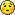All times are UTC+02:00

 Post new topic  Reply to topic Page 1 of 1 [ 1 post ]
 Print view Previous topic | Next topic
Author Message
 Post subject: REAPER with project sample rates higher than 192khzPostPosted: Sun Mar 15, 2020 3:27 pm

Joined: Sat Feb 21, 2015 10:53 pm
Posts: 16
Good Morning!

OK, so I know this is a complete outlier case but here it goes:

If I want to use the DAW Reaper in a project, and if a plugin does not support oversampling, I might set the project sample rate anywhere from 384Khz (8x oversampled from 48khz) to 1.536Mhz (32x oversampled from 48khz).

However, when I go above 192khz, the project will crash if Steretool is in the chain - I assume because it doesn't support input sampling rates over 192khz. Would it be possible to support such high sample rates for these outlier cases without first downsampling? I imagine part of that would have to involve raising the FFT window option to more samples than the 4096 maximum since:

44.1Khz / 4096 = 10.77 Hz bins
48Khz / 4096 = 11.71 Hz bins
96Khz / 4096 = 23.44 Hz bins (so we would need FFT window size of 8192 samples to reach the same level of 11.71Hz bin granularity)
192Khz / 4096 = 46.88 Hz bins (so we would need FFT of 16384 to reach the same level of granularity)
384Khz / 4096 = 93.75 Hz bins (so we would need FFT of 32768 to reach the same level of granularity)
768Khz / 4096 = 187.5 Hz bins (so we would need FFT of 65536 to reach the same level of granularity)
1,536Khz / 4096 = 375 Hz bins (so we would need FFT of 131072 to reach the same level of granularity)

Of course, basing it on the usual standard of 44.1Khz,

44.1Khz / 4096 = 10.77 Hz bins
48Khz / 4096 = 11.72 Hz bins (so we would need FFT window size of 4464 samples to reach the same level of 10.77Hz granularity)
96Khz / 4096 = 23.44 Hz bins (so we would need FFT of 8928 to reach the same level of granularity)
192Khz / 4096 = 46.88 Hz bins (so we would need FFT of 17840 to reach the same level of granularity)
384Khz / 4096 = 93.75 Hz bins (so we would need FFT of 35680 to reach the same level of granularity)
768Khz / 4096 = 187.5 Hz bins (so we would need FFT of 71344 to reach the same level of granularity)
1,536Khz / 4096 = 375 Hz bins (so we would need FFT of 142672 to reach the same level of granularity)

Of course, for the truly insane who might want to process DSD

Based on 44.1Khz at 4096 samples for FFT bin of 10.77Hz (resolution for, say EQ):

DSD 64 = 2.8Mhz / 4096 = 689.06 Hz bins (so we would need FFT window size of 262144 samples to reach the same level of granularity)
DSD 128 = 5.6Mhz / 4096 = 1378.13 Hz bins (so we would need FFT of 524288 to reach the same level of granularity)
DSD 256 = 11.3Mhz / 4096 = 2756.25 Hz bins (so we would need FFT of 1048576 to reach the same level of granularity)
DSD 512 = 22.6Mhz / 4096 = 5512.5 Hz bins (so we would need FFT of 2097152 to reach the same level of granularity)
DSD 1024 = 45.2Mhz / 4096 = 11025 Hz bins (so we would need FFT of 4194304 to reach the same level of granularity)

Based on 48Khz at 4096 samples for FFT bin of 11.72Hz (resolution for, say EQ):

DSD 64 = 2.8Mhz / 4096 = 689.06 Hz bins (so we would need FFT of 240848 to reach the same level of granularity)
DSD 128 = 5.6Mhz / 4096 = 1378.13 Hz bins (so we would need FFT of 481696 to reach the same level of granularity)
DSD 256 = 11.3Mhz / 4096 = 2756.25 Hz bins (so we would need FFT of 963392 to reach the same level of granularity)
DSD 512 = 22.6Mhz / 4096 = 5512.5 Hz bins (so we would need FFT of 1926768 to reach the same level of granularity)
DSD 1024 = 45.2Mhz / 4096 = 11025 Hz bins (so we would need FFT of 3853520 to reach the same level of granularity)

Anyway, apologies for the rant, I got excitedTopDisplay posts from previous: All posts1 day7 days2 weeks1 month3 months6 months1 year Sort by AuthorPost timeSubject AscendingDescending
 Post new topic  Reply to topic Page 1 of 1 [ 1 post ]

 All times are UTC+02:00

 You cannot post new topics in this forumYou cannot reply to topics in this forumYou cannot edit your posts in this forumYou cannot delete your posts in this forumYou cannot post attachments in this forum

Search for:
 Jump to:  Select a forum ------------------ Stereo Tool    News    Links to other media Help    Stereo Tool installation troubles    Stereo Tool and AM/FM transmitters    Hardware selection    Sound cards    3rd party software    Getting the best sound    Can you listen to my stream?    Other questions Feedback    Use cases    Bug reports    Wish list Settings repository    Share your Stereo Tool settings    Language/Translation files    Skins Other language forums    Nederlandstalig forum (Dutch language forum)    Deutschsprachiges Forum (German language forum)    Forum en français (French language forum)    Foro en español (Spanish language forum)    Italian section